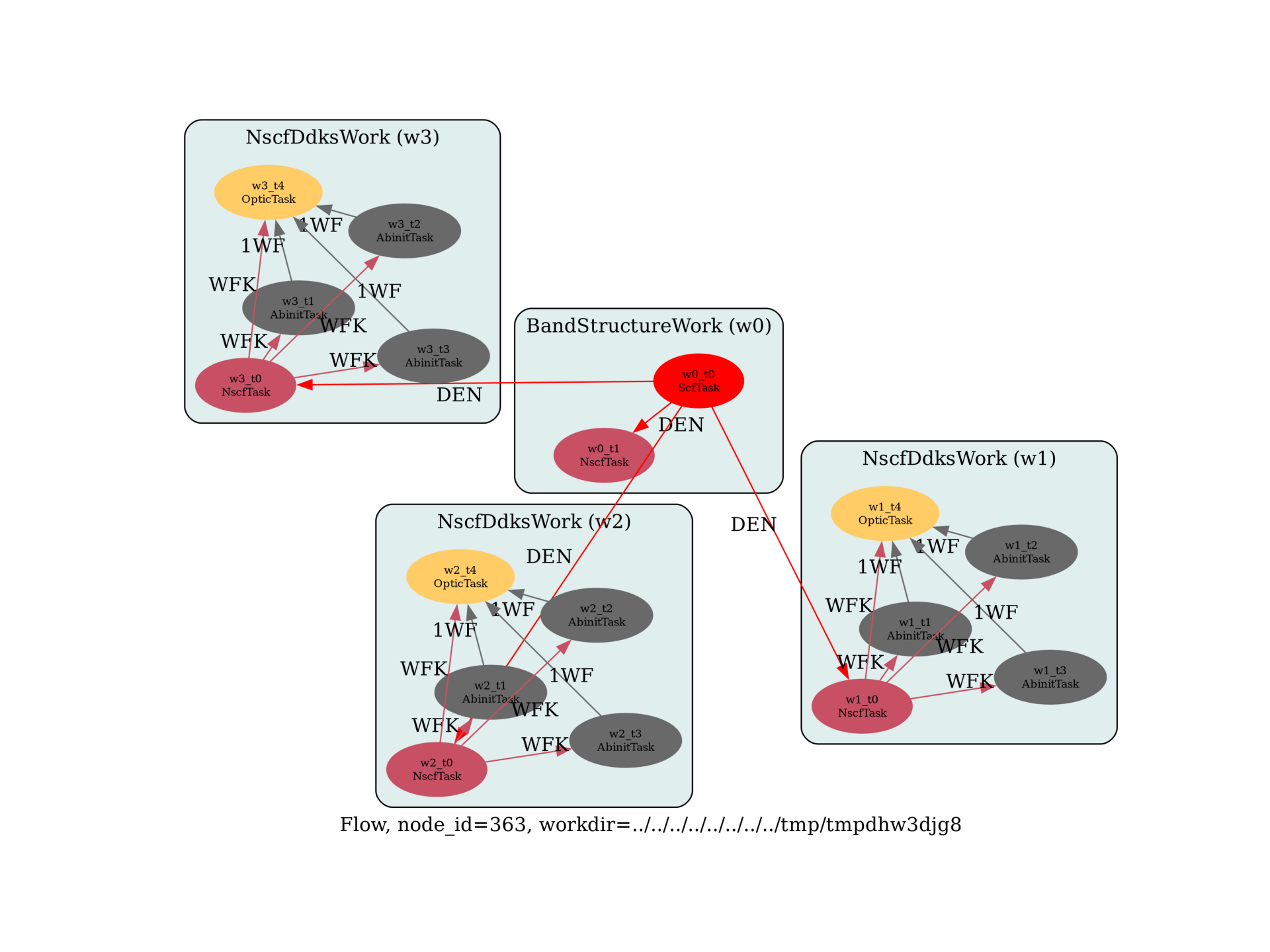Optic Flow¶

This example shows how to create a flow to compute optical spectra with Optic (independent particle approximation, no local field effects) and perform a convergence study with respect to the k-point sampling.

import sys
import os
import abipy.data as abidata
import abipy.abilab as abilab
import abipy.flowtk as flowtk

def build_flow(options, paral_kgb=0):
"""
Build flow for the calculation of optical properties with optic + band structure
along high-symmetry k-path. DDK are computed with 3 k-meshes of increasing density
to monitor the convergece of the spectra.
"""
# Working directory (default is the name of the script with '.py' removed and "run_" replaced by "flow_")
if not options.workdir:
options.workdir = os.path.basename(sys.argv).replace(".py", "").replace("run_", "flow_")

multi = abilab.MultiDataset(structure=abidata.structure_from_ucell("GaAs"),
pseudos=abidata.pseudos("31ga.pspnc", "33as.pspnc"), ndtset=2)

# Usa same shifts in all tasks.
shiftk = [[0.5, 0.5, 0.5],
[0.5, 0.0, 0.0],
[0.0, 0.5, 0.0],
[0.0, 0.0, 0.5]]

# Global variables.
multi.set_vars(ecut=2, paral_kgb=paral_kgb)

# Dataset 1 (GS run)
multi.set_vars(tolvrs=1e-8, nband=4)
multi.set_kmesh(ngkpt=[4, 4, 4], shiftk=shiftk)

# NSCF run on k-path with large number of bands
multi.set_vars(iscf=-2, nband=20, tolwfr=1.e-9)
multi.set_kpath(ndivsm=10)

# Initialize the flow.
flow = flowtk.Flow(options.workdir, manager=options.manager)

# GS to get the density + NSCF along the path.
scf_inp, nscf_inp = multi.split_datasets()
bands_work = flowtk.BandStructureWork(scf_inp, nscf_inp)
flow.register_work(bands_work)

# Build OpticInput used to compute optical properties.
optic_input = abilab.OpticInput(
broadening=0.002,          # Value of the smearing factor, in Hartree
domega=0.0003,             # Frequency mesh.
maxomega=0.3,
scissor=0.000,             # Scissor shift if needed, in Hartree
tolerance=0.002,           # Tolerance on closeness of singularities (in Hartree)
num_lin_comp=2,            # Number of components of linear optic tensor to be computed
lin_comp=(11, 33),         # Linear coefficients to be computed (x=1, y=2, z=3)
num_nonlin_comp=2,         # Number of components of nonlinear optic tensor to be computed
nonlin_comp=(123, 222),    # Non-linear coefficients to be computed
)

# ddk_nband is fixed here, in principle it depends on nelect and the frequency range in chi(w).
ddk_nband = 20

# Perform converge study wrt ngkpt (shiftk is constant).
ngkpt_convergence = [[4, 4, 4], [8, 8, 8], [16, 16, 16]]

from abipy.flowtk.dfpt_works import NscfDdksWork
for ddk_ngkpt in ngkpt_convergence:
# Build work for NSCF from DEN produced by the first GS task + 3 DDKs.
# All tasks use more bands and a denser k-mesh defined by ddk_ngkpt.
ddks_work = NscfDdksWork.from_scf_task(bands_work, ddk_ngkpt, shiftk, ddk_nband)
flow.register_work(ddks_work)

# Build optic task to compute chi with this value of ddk_ngkpt.

return flow

# This block generates the thumbnails in the AbiPy gallery.
# You can safely REMOVE this part if you are using this script for production runs.
__name__ = None
import tempfile
options = flowtk.build_flow_main_parser().parse_args(["-w", tempfile.mkdtemp()])
build_flow(options).graphviz_imshow()

@flowtk.flow_main
def main(options):
"""
This is our main function that will be invoked by the script.
flow_main is a decorator implementing the command line interface.
Command line args are stored in `options`.
"""
return build_flow(options)

if __name__ == "__main__":
sys.exit(main())Run the script with:

run_optic.py -s

then use:

abirun.py flow_optic robot optic

to create a robot for OPTIC.nc files. Then inside the ipytho shell type:

In : %matplotlib
In : robot.plot_linopt_convergence()Total running time of the script: ( 0 minutes 0.829 seconds)

Gallery generated by Sphinx-Gallery